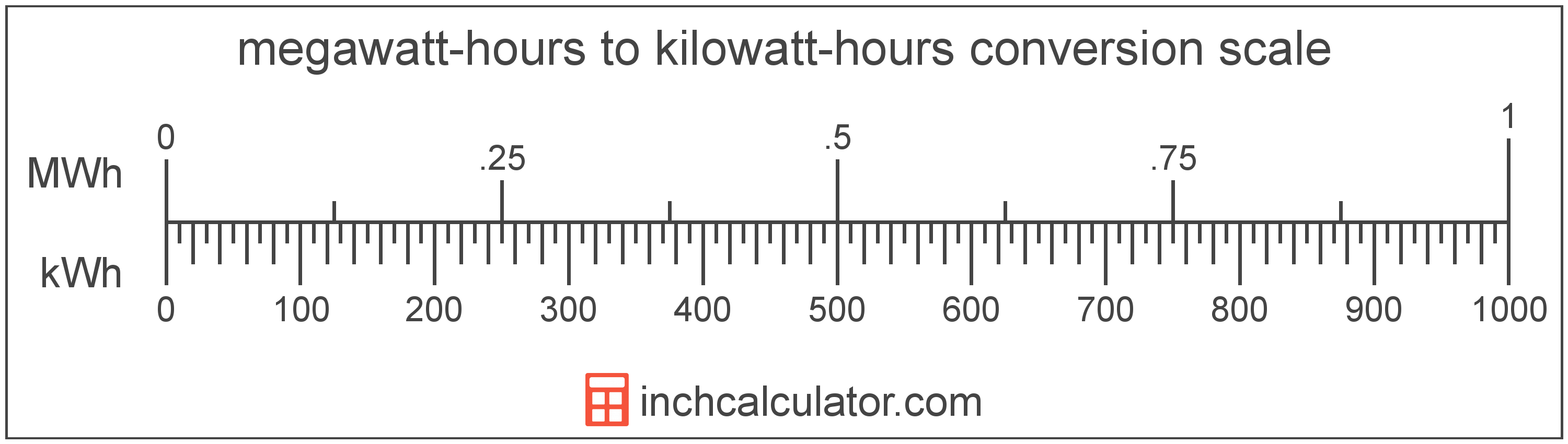# Megawatt-Hours to Kilowatt-Hours Converter

Enter the energy in megawatt-hours below to get the value converted to kilowatt-hours.

Results in Kilowatt-Hours:1 MWh = 1,000 kWh

Do you want to convert kilowatt-hours to megawatt-hours?

## How to Convert Megawatt-Hours to Kilowatt-Hours

To convert a measurement in megawatt-hours to a measurement in kilowatt-hours, multiply the energy by the following conversion ratio: 1,000 kilowatt-hours/megawatt-hour.

Since one megawatt-hour is equal to 1,000 kilowatt-hours, you can use this simple formula to convert:

kilowatt-hours = megawatt-hours × 1,000

The energy in kilowatt-hours is equal to the energy in megawatt-hours multiplied by 1,000.

For example, here's how to convert 5 megawatt-hours to kilowatt-hours using the formula above.
kilowatt-hours = (5 MWh × 1,000) = 5,000 kWh### How Many Kilowatt-Hours Are in a Megawatt-Hour?

There are 1,000 kilowatt-hours in a megawatt-hour, which is why we use this value in the formula above.

1 MWh = 1,000 kWh

## What Is a Megawatt-Hour?

A megawatt-hour is a measure of electrical energy equal to one megawatt, or 1,000,000 watts, of power over a one hour period. Megawatt-hours are a measure of electrical work performed over a period of time, and are often used as a way of measuring energy usage by electric companies.

Megawatt-hours are usually abbreviated as MWh, although the formally adopted expression is MW·h. The abbreviation MW h is also sometimes used. For example, 1 megawatt-hour can be written as 1 MWh, 1 MW·h, or 1 MW h.

In formal expressions, the centered dot (·) or space is used to separate units used to indicate multiplication in an expression and to avoid conflicting prefixes being misinterpreted as a unit symbol.

## What Is a Kilowatt-Hour?

A kilowatt-hour is a measure of electrical energy equal to one kilowatt, or 1,000 watts, of power over a one hour period. Kilowatt-hours are a measure of electrical work performed over a period of time, and are often used as a way of measuring energy usage by electric companies.

Kilowatt-hours are usually abbreviated as kWh, although the formally adopted expression is kW·h. The abbreviation kW h is also sometimes used. For example, 1 kilowatt-hour can be written as 1 kWh, 1 kW·h, or 1 kW h.

## Megawatt-Hour to Kilowatt-Hour Conversion Table

Table showing various megawatt-hour measurements converted to kilowatt-hours.
Megawatt-hours Kilowatt-hours
0.001 MWh 1 kWh
0.002 MWh 2 kWh
0.003 MWh 3 kWh
0.004 MWh 4 kWh
0.005 MWh 5 kWh
0.006 MWh 6 kWh
0.007 MWh 7 kWh
0.008 MWh 8 kWh
0.009 MWh 9 kWh
0.01 MWh 10 kWh
0.02 MWh 20 kWh
0.03 MWh 30 kWh
0.04 MWh 40 kWh
0.05 MWh 50 kWh
0.06 MWh 60 kWh
0.07 MWh 70 kWh
0.08 MWh 80 kWh
0.09 MWh 90 kWh
0.1 MWh 100 kWh
0.2 MWh 200 kWh
0.3 MWh 300 kWh
0.4 MWh 400 kWh
0.5 MWh 500 kWh
0.6 MWh 600 kWh
0.7 MWh 700 kWh
0.8 MWh 800 kWh
0.9 MWh 900 kWh
1 MWh 1,000 kWh

## References

1. Bureau International des Poids et Mesures, The International System of Units (SI), 9th edition, 2019, https://www.bipm.org/documents/20126/41483022/SI-Brochure-9-EN.pdf